# Pandaral·lel

``````# Standard apply

df.apply(func)``````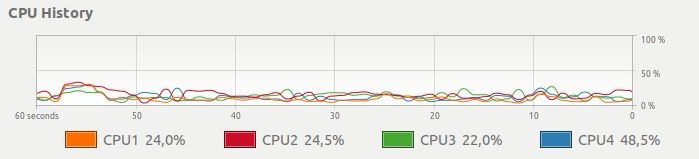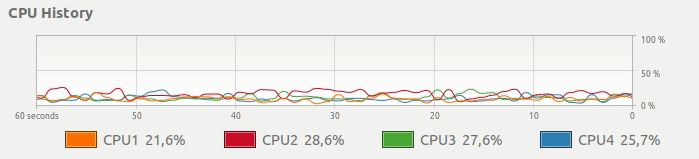Pandaral·lel 如何帮助解决这个问题？

Pandaral·lel 的想法是将pandas计算分布在计算机上所有可用的CPU上，以显着提高速度。

## 安装

`pip install pandarallel`

## 要求

• Linux或macOS（目前不支持Windows）

## 警告

• 并行化有成本（实现新进程，通过共享内存发送数据等等），因此只有并行化的计算量足够高时，并行化才有效。对于非常少量的数据，使用并行并不总是值得的。

## 基准

• 操作系统：Linux Ubuntu 16.04
• 硬件：Intel Core i7 @ 3.40 GHz - 4核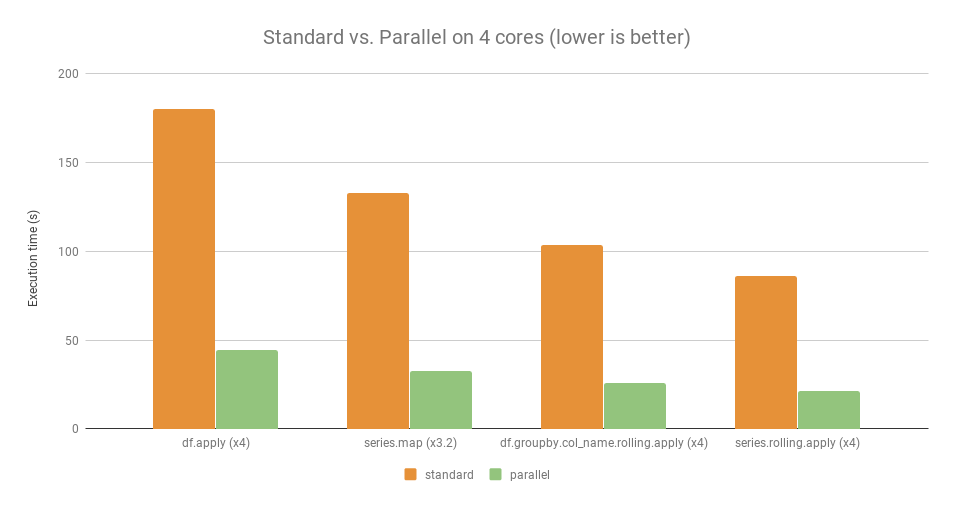## API

``import pandarallel``

``pandarallel.initialize()``

• `shm_size_mb`：Pandarallel共享内存的大小（MB）。如果默认值太小，则可以设置较大的值。默认情况下，它设置为2 GB。（INT）
• `nb_workers`：工人数量。默认情况下，它设置为操作系统看到的核心数。（INT）
• `progress_bar`：将其设置`True`为显示进度条。
• `verbose`：详细程度。> 1显示所有日志 - 1，仅显示初始化日志 - <1显示无日志（int）

`df.apply(func)` `df.parallel_apply(func)`
`df.applymap(func)` `df.parallel_applymap(func)`
`df.groupby(args).apply(func)` `df.groupby(args).parallel_apply(func)`
`df.groupby(args1).col_name.rolling(args2).apply(func)` `df.groupby(args1).col_name.rolling(args2).parallel_apply(func)`
`series.map(func)` `series.parallel_map(func)`
`series.apply(func)` `series.parallel_apply(func)`
`series.rolling(args).apply(func)` `series.rolling(args).parallel_apply(func)`

## 故障排除

Ubuntu上，您可以获得核心数量`\$ grep -m 1 'cpu cores' /proc/cpuinfo`

Pandaral·lel：在多CPU环境简单高效并行运行Pandas

Pandaral·lel :github

# Modi

pandas库是python中最有名的数据分析库，因为dataframe这种易用强大的数据类型，pandas成为数据科学必备套件。pandas可以和很多包联合使用，比如与机器学习sklearn、统计分析statsmodels、可视化searborn&matplotlib等等。下图是近几年python包的使用量趋势，pandas一骑绝尘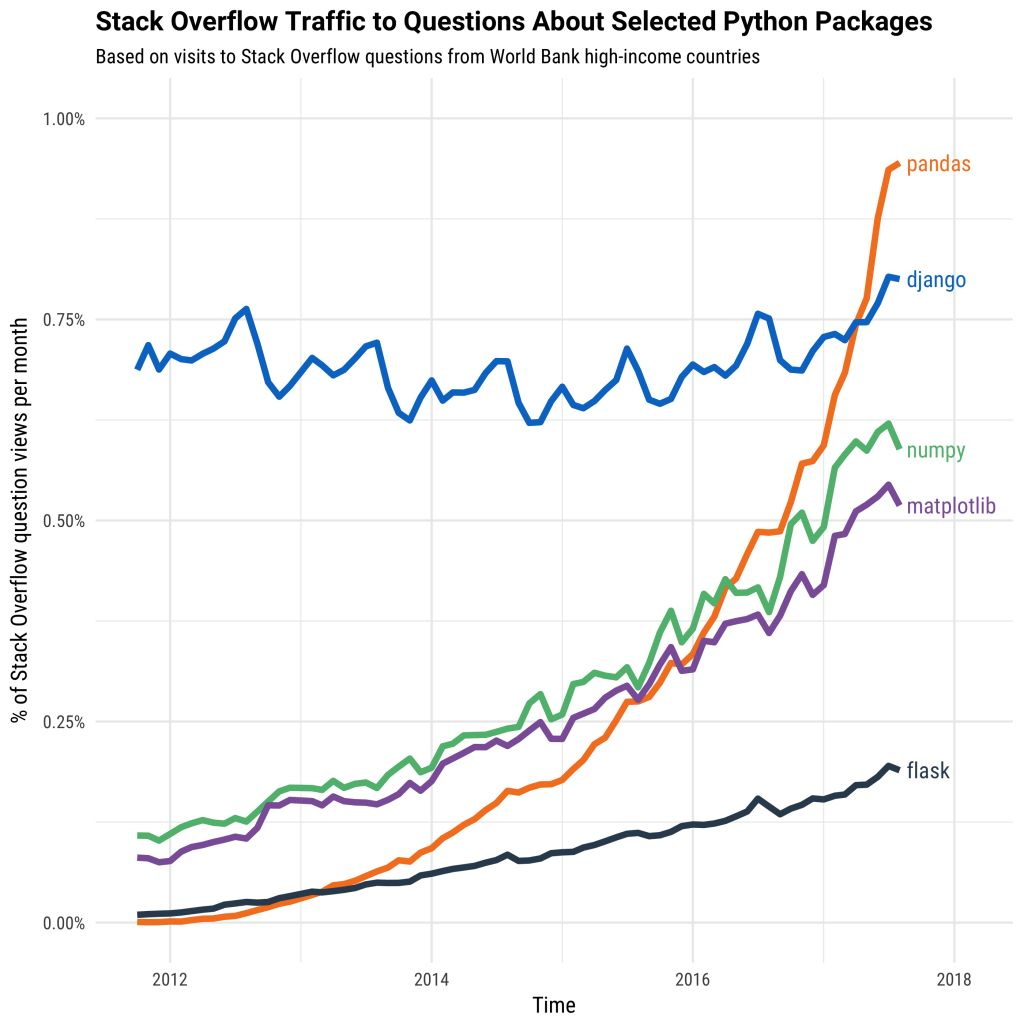pandas默认只调用电脑单个的CPU进行数据读取和运算，但是当前的电脑大多是4核，甚至8核，而如果常规使用pandas意味着我们没有充分使用电脑的性能。

### 安装

``````pip3 install ray
pip3 install modin``````

### modin使用

modin的用法与pandas相差无几，只是在导入的时候略有不同。

``import modin.pandas as pd``

### 读取速度

``````import time
import pandas as pd

start = time.time()
end = time.time()
print(end-start)``````
``1.611081838607788``
``pandas导入107M的test.csv耗时1.78s``
``````import time
import modin.pandas as pd

start = time.time()
#test.csv 107M
end = time.time()
print(end-start)``````
``0.7474761009216309``
``modin只用了0.75s，加快了2.1倍。``

### 运算速度

pd.concat是用来连接多个dataframe的操作函数，当我们的df很大时，pd.concat就会变慢。我们在这里实验一下pandas和modin各自的速度

``````import time
import pandas as pd

start = time.time()
newdf = pd.concat([df for _ in range(100)])
end = time.time()
print(end-start)``````
``10.625022888183594``
``````import time
import modin.pandas as pd

start = time.time()
newdf = pd.concat([df for _ in range(100)])
end = time.time()
print(end-start)``````
``0.7488729953765869``
``同样的pd.concat操作，modin比pandas快了14倍。我又做了几个对比，制作成表格``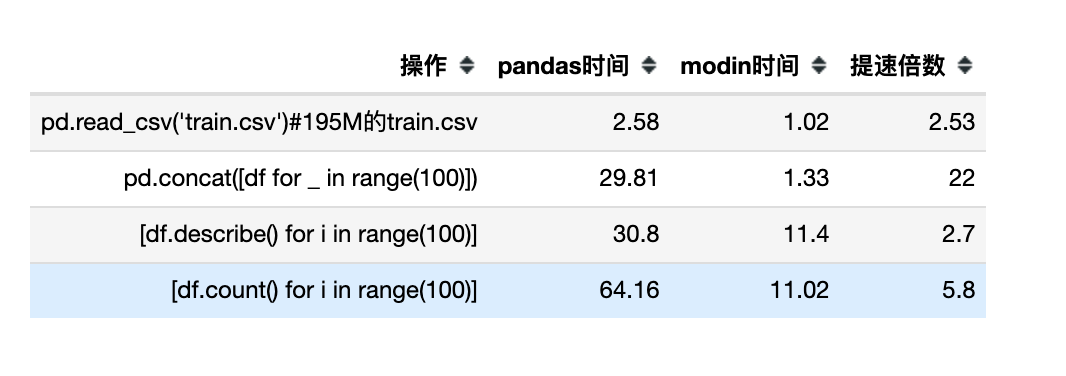### 实战技巧

modin目前仍然正在发展中，并不是所有的pandas的函数都能加速。如果加速遇到报错，说明这个操作modin不支持。

``````import ray
import warnings
warnings.filterwarnings('ignore')
ray.init(num_cpus=4, ignore_reinit_error=True)
import modin.pandas as pd``````

``````import os
os.environ["MODIN_OUT_OF_CORE"]='true'
import modin.pandas as pd``````Modin是为加速pandas而开发的包，可以自动按照电脑CPU数调整运行的核数。